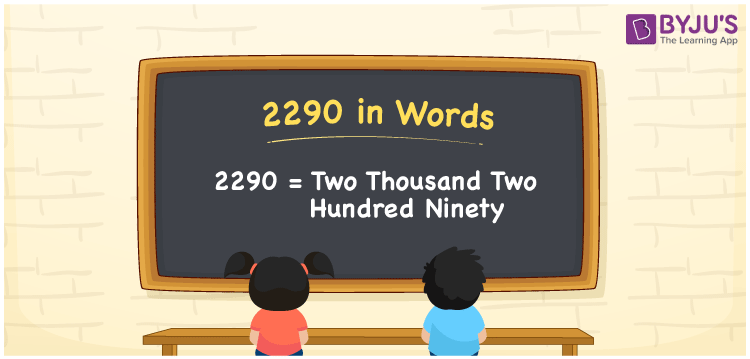# 2290 in Words

We can write 2290 in words as Two thousand two hundred ninety. It is possible to convert the given number into words with the help of a place value chart. Also, we know that we use the word form of cardinal numbers in general discussions. Suppose you purchased a wall paint that costs Rs. 2290, you can say that “I purchased a wall paint for Two thousand two hundred ninety rupees”. Thus, we use the word form of 2290 to express it to others.

 2290 in words Two thousand two hundred ninety Two thousand two hundred ninety in Numbers 2290

## 2290 in English Words

Using the English alphabet, we can express numbers in words. So, we can spell 2290 in words as “Two thousand two hundred ninety.## How to Write 2290 in Words?

In this section, you will learn how to write the number 2290 using a place value chart. The below table shows the place value for each digit of the number 2290.

 Thousands Hundreds Tens Ones 2 2 9 0

Here, ones = 0, tens = 9, hundreds = 2, and thousands = 2.

Thus, we can write the expanded form as:

2 × Thousand + 2 × Hundred + 9 × Ten + 0 × One

= 2 × 1000 + 2 × 100 + 9 × 10 + 0 × 1

= 2000 + 200 + 90

= Two thousand + Two hundred + Ninety

= Two thousand two hundred ninety

Therefore, 2290 in words = Two thousand two hundred ninety.

Learn in detail about place value here.

2290 is a natural number that precedes 2291 and succeeds 2289.

2290 in words – Two thousand two hundred ninety

Is 2290 an odd number? – No

Is 2290 an even number? – Yes

Is 2290 a prime number? – No

Is 2290 a composite number? – Yes

Is 2290 a perfect square number? – No

Is 2290 a perfect cube number? – No

## Frequently Asked Questions on 2290 in Words

Q1

### What number is Two thousand two hundred ninety in words?

The number 2290 can be expressed as Two thousand two hundred ninety in words.
Q2

### How to write an amount of Rs. 2290 in words on a cheque?

On a cheque, we can write the amount of Rs. 2290 in words as “Two thousand two hundred ninety rupees only”.
Q3

### Express the value of 2290 + 13740 in words.

2290 + 13740 = 16030 Therefore, the value of 2290 + 13740, i.e. 16030 in words is Sixteen thousand and thirty.